SearchMatrix addition is fairly simple, and is done entry-wise.

•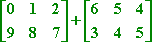I need to add the pairs of entries, and then simplify for the final answer: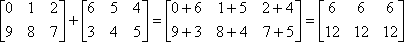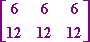Up until now, you've been able to add any two things you felt like: numbers, variables, equations, and so forth. But addition doesn't always work with matrices.

• Perform the indicated operation, or explain why it is not possible.
•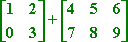Since matrices are added entry-wise, I have to add the 1 and the 4, the 2 and 5, the 0 and the 7, and the 3 and the 8. But what do I add to the 6 and to the 9? There are no corresponding entries in the first matrix that can be added to these entries in the second matrix. So the answer is:

I can't add these matrices, because they're not the same size.

This is always the case: To be able to add two matrices, they must be of the same size. If they are not the same size (if they do not have the same "dimensions"), then the addition is "not defined" (doesn't make mathematical sense).

• Given the following matrices, find A  Band A  C, or explain why you can not.
•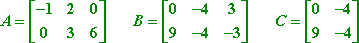A and B are the same size, each being 2 × 3 matrices, so I can subtract, working entry-wise: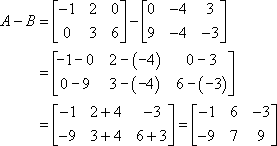However, A and C are not the same size, since A is 2 × 3 and C is 2 × 2. So this subtraction is not defined.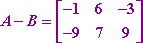A  C is not defined, because A and C are not the same size.

Matrix addition and subtraction, where defined (that is, where the matrices are the same size so addition and subtraction make sense), can be turned into homework problems.

• Find the values of xand y given the following equation:
•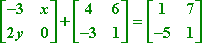First, I'll simplify the left-hand side a bit by adding entry-wise: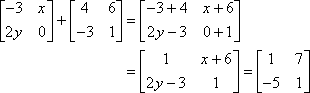Since matrix equality works entry-wise, I can compare the entries to create simple equations that I can solve. In this case, the 1,2-entries tell me that x + 6 = 7, and the 2,1-entries tell me that 2y  3 = 5. Solving, I get:

x + 6 = 7
x = 1

2y  3 = 5
2y = 2

y = 1